• ## c++学生信息管理系统

千次阅读 多人点赞 2020-02-19 10:33:34
c++学生信息管理系统 引言 这是我写的第一篇博客，是关于一个小的c艹课程设计，其中还有许多不足的地方，例如我使用的是UTF-8编码，但输出中文还是会有乱码的情况；还有就是从文件读出和存入是无法控制格式，以及我...
c++学生信息管理系统
引言
这是我写的第一篇博客，是关于一个小的c艹课程设计，其中还有许多不足的地方，例如我使用的是UTF-8编码，但输出中文还是会有乱码的情况；还有就是从文件读出和存入是无法控制格式，以及我是用的是面向对象的方式但是感觉很多地方都没有体现出OPP的精髓，希望大家能帮我指出错误
目录
摘要
一、需求分析：
1.1概要
1.2功能要求
二、总体设计
2.1主要功能
2.2定义类和函数
2.3程序功能结构图
三、详细设计
3.1函数功能介绍
四、测试结果展示
五、软件使用说明书
六、总结
6.1错误总结
6.2学习总结
七、参考资料
八、开发工具
九、源代码
一、需求分析
1.1 概要：
学生信息管理的需要，建立一个“学生信息管理系统”，以方便对学校信息数据的录入、修改、删除、查询等一系列功能。
1.2功能要求：
（1）有菜单界面并且可以对功能进行选择；
（2）能录入姓名、性别、学号、成绩（数学、英语、c++、总成绩、平均成绩）；
（3）按成绩对学生信息进行排序；
（4）按姓名对学生信息进行修改；
（5）按姓名对学生信息进行删除；
（6）按姓名或学号对学生信息进行查询；
二、总体设计
2.1主要功能：
（1）	从键盘录入学生个人信息并保存到文件中；
（2）	从文件中读取学生个人信息；
（3）	按学号对学生信息进行查找；
（4）	按姓名对学生信息进行查找；
（5）	按姓名对学生信息进行修改；
（6）	按姓名对学生信息进行删除；
（7）	计算各项成绩的平均成绩以及及格率；
2.2定义类和函数：
（1）	主函数main（）：调用各项实现函数对学生信息进行处理；
（2）	定义类Student：用于定义成员结构体；
a)	Input（）函数提示输入信息并进行输入；
c)	Show1（）函数为Display1（）函数定义输出格式；
d)	Show2（）函数为Display2（）函数定义输出格式；
（3）	定义类Studentmassage：用于实现各项功能函数；
a)	构造函数Studentmassage（）：构建链表，判断文件中的信息是否为空，并入文件中的信息；
b)	析构函数~Studentmassage（）：清空当前文件所有信息；
d)	查找函数Find（）：通过学生姓名或学号查找特定学生信息；
e)	修改函数ModifyItem（）：通过姓名对特定学生的信息进行修改；
f)	删除函数RemoveItem（）；通过姓名删除特定学生的信息；
g)	记录函数ListCount（）：记录建立链表的数目及当前学生总人数；
h)	交换函数Swap（）：排序时对两学生信息进行交换；
i)	排序函数Sort（）：成绩对学生信息进行排序；
j)	保存函数Save（）：将新建信息存入文件中；
l)	输出信息Display1（）：输出链表中的各项信息；
m)	输出信息Display1（）：输出链表中的各项信息；
n)	输出信息Displa12（）：输出排序后的总成绩和排名；
o)	计算函数Average（）：计算各项成绩的平均成绩和及格率并根据用户需求输出；
2.3程序功能结构图：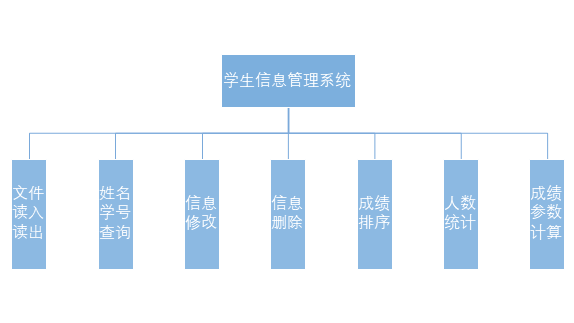三、详细设计
3.1函数功能介绍：
流程图如下：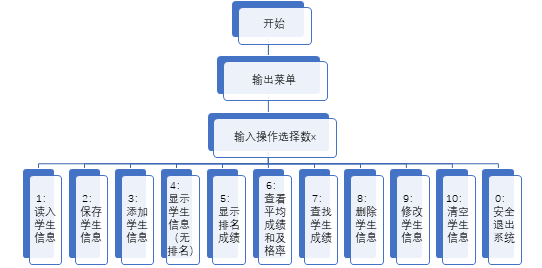函数实现代码：
Studentmassage::Studentmassage()		//构造函数
{
in.open("sort.txt");
if (!in)	cout << "这是一个新系统，无学生信息，请先输入。" << endl;
else
{
while (!in.eof())
{
if (end->name == '\0')	break;
end->Next = new Student;
end = end->Next;
}
in.close();
cout << "\t\t 读取学生信息成功！" << endl;
}
}

流程图如下：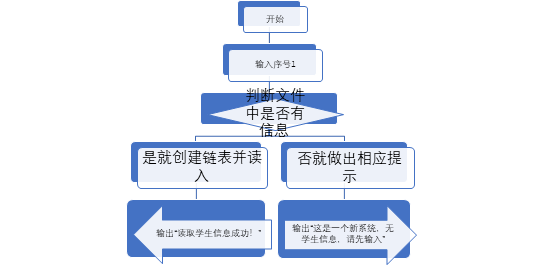（3）	保存学生信息：Studentmassage类的Save（）函数找到相应文件，将当前链表中的所有信息都存入文件中；
函数实现代码：
void Studentmassage::Save()
{
out.open("sort.txt");
for (Student *p = head->Next; p != end; p = p->Next)
{
out << p->name << "\t" << p->ID << "\t" << p->CPP << "\t" << p->MATH << "\t" << p->ENGLISH << "\t" << p->sum << p->rank << "\t" << endl;
out.close();
}
}

流程图如下：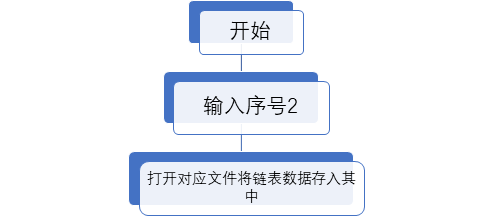函数实现代码：
void Studentmassage::AddItem()
{
end->Input();
//	scpp += end->CPP;
//	smath += end->MATH;
//	seng += end->ENGLISH;
end->Next = new Student;
end = end->Next;
cout << "添加成功！" << endl;
system("pause");
//	cout << "请输入任意字符！继续......";
//	getchar();
}

（5）	显示信息（不含排名）：通过Studentmassage类的Display1（）函数调用Student类的Show1（）函数显示最初始未经排名的信息；
函数实现代码：
void Studentmassage::Display1()
{
cout << "姓名: \t\t" << "性别: \t\t" << "学号: \t\t"
<< "C++: \t" << "数学: \t" << "英语：\t"
<< " 总成绩: \t" << "\n\n";
for (Student*p = head->Next; p != end; p = p->Next)
p->Show1();
system("pause");
//	cout << "输入任意字符！继续······";
//	getchar();
}

（6）	显示成绩（含排名）：通过Studentmassage类的Display2（）函数先调用同类的Sort（）函数进行排序并添加排名信息，再调用Student类的Show2（）函数进行信息输出；
函数实现代码：
void Studentmassage::Display2()
{
Sort();
cout << “姓名: \t\t” << “性别: \t\t” << “学号: \t\t”
<< " 总成绩: \t" << "排名: " << “\n\n”;
for (Student*p = head->Next; p != end; p = p->Next)
p->Show2();
system(“pause”);
//	cout << “输入任意字符！继续······”;
//	getchar();
}
（7）	查看平均成绩和及格率：Studentmassage类的Average（）函数中先创建一个Student类的指针p，用来遍历链表，算出各项成绩的总和并统计不及格人数，通过调用ListCount（）函数获得链表个数即当前总人数；
函数实现代码：
void Studentmassage::Average()
{
scpp = 0.0, smath = 0.0, seng = 0.0, ssum = 0.0;
int c = 0, m = 0, e = 0;	//记录各科不及格人数
Student *p;
for (p = head->Next; p->Next != end; p = p->Next)
{
scpp += p->CPP; smath += p->MATH; seng += p->ENGLISH; ssum += p->sum;
if (p->CPP < 60) c++;
if (p->MATH < 60) m++;
if (p->ENGLISH < 60) e++;
//		cout << p->CPP << endl;
}
scpp += p->CPP; smath += p->MATH; seng += p->ENGLISH; ssum += p->sum;
int num = Studentmassage::ListCount();
//	cout << num << endl;
double avcpp = scpp / num;			//c++平均成绩
double avmath = smath / num;		//数学平均成绩
double aveng = seng / num;			//英语平均成绩
double avsum = ssum / num;			//总分平均成绩
double procpp = (num - c) / num;	//c++及格率
double promath = (num - m) / num;			//数学及格率
double proeng = (num - e) / num;			//英语及格率
//	cout << scpp << "\t" << smath << "\t" << seng << "\t" << ssum << "\n";
cout << "\n\t\t*********************************\n";
cout << "\t\t※ 0.输出各项平均成绩\n";
cout << "\t\t※ 1.输出c++平均成绩 \n\t\t※ 2.输出数学平均成绩 \n";
cout << "\t\t※ 3.输出英语平均成绩 \n\t\t※ 4.输出总平均成绩 \n";
cout << "\t\t※ 5.输出各科及格率";
cout << "\n\t\t*********************************\n\t\t请输入相应序号进行选择： ";
int x; cin >> x;
switch (x)
{
case 0: {
cout << "\t\tc++平均成绩\t" << "数学平均成绩\t" << "英语平均成绩\t" << "总平均成绩\t\n";
cout << "\t\t" << avcpp << "\t\t" << avmath << "\t\t" << aveng << "\t\t" << avsum << endl; break;
}
case 1:cout << "\t\tc++平均成绩 : " << avcpp << endl; break;
case 2:cout << "\t\t数学平均成绩 : " << avmath << endl; break;
case 3:cout << "\t\t英语平均成绩 : " << aveng << endl; break;
case 4:cout << "\t\t总平均成绩 : " << avsum << endl; break;
case 5: {
cout << "\t\tc++及格率\t" << "数学及格率\t" << "英语及格率\t" << endl;
cout << "\t\t" << procpp * 100 << "%" << "\t\t" << promath * 100 << "%"
<< "\t\t" << proeng * 100 << "%" << "\t\t" << endl; break;
}
}
system("pause");
}

流程图如下：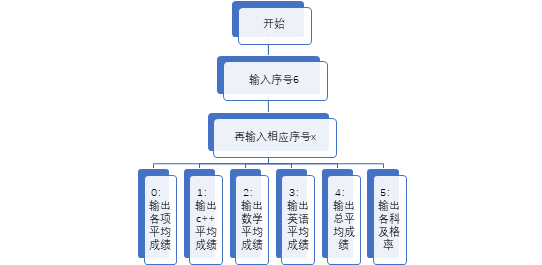（8）	查找学生信息：通过FindItem（）和FindID（）函数判断是否有相对应名字或学号的学生；
函数实现代码：
void Studentmassage::Find()
{
char name, ID;
int x;
Student *p = NULL;
cout << "\n\t\t*********************************\n";
cout << "\t\t※ 1.按学生的姓名查找 \n\t\t※ 2.按学生学号查找 ";
cout << "\n\t\t*********************************\n 请选择： ";
cin >> x;
switch (x)
{
case 1:
{
cout << "\t\t 请输入要查找学生姓名：  ";
cin >> name;
if (p = FindItem(name))
{
p->Next->Show1();
system("pause");
//			cout << "输入任意字符！继续······ \n";
//			getchar();
}
else
{
cout << "\t\t 没有找到相匹配的学生！  " << "\n\n";
system("pause");
//			cout << "请输入任意字符！继续······";
//			getchar();
}
} break;
case 2:
{
cout << "\t\t 请输入要查找的学生的学号： "; cin >> ID;
if (p = FindID(ID))
{
p->Next->Show1();
system("pause");
//			cout << " 输入任意字符！继续…… ";
//			getchar();
}
else
{
cout << "\t\t 没有找到相匹配的学生！ " << '\n' << endl;
system("pause");
//			cout << " 输入任意字符！继续…… ";
//			getchar();
}
} break;
}
}

流程图如下：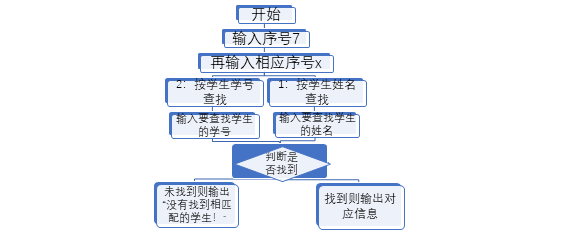（9）	删除学生信息：Studentmassage类的RemoveItem（）函数通过调用FindItem（）函数查找是否有相对应的名字，有则删除该段链表并输出“删除成功！”，没有则输出“没有找到！”。
函数实现代码：
void Studentmassage::RemoveItem()
{
char name;
Student *p = NULL, *temp = NULL;
cout << "\t\t 请输入要删除学生的姓名 ：" << endl; cin >> name;
if (p = FindItem(name))
{
temp = p->Next;
p->Next = p->Next->Next;
delete temp;
cout << "\t\t 删除成功！" << endl;
system("pause");
//		cout << "输入任意字符！继续······";
//		getchar();
}
else
{
cout << "\t\t 没有找到！" << endl;
system("pause");
//		cout << "输入任意字符！继续······";
//		getchar();
}
}

					流程图如下：

（10）	修改学生信息：Studentmassage类ModifyItem（）函数通过FindItem（）函数查找是否有相对应的名字，有则按提示步骤操作，没有则输出“没有找到”；
函数实现代码：
void Studentmassage::ModifyItem()
{
char name;
Student *p = NULL;
cout << "\t\t 请输入需要修改人的姓名 ："; cin >> name;
if (p = FindItem(name))
{
cout << "\t\t 已找到该学生信息，请按步骤输入新的信息！" << endl;
p->Next->Input();
cout << "修改成功！ " << endl;
system("pause");
//		cout << "请输入任意字符！继续······";
//		getchar();
}
else
{
cout << "\t\t 没有找到！" << endl;
system("pause");
//		cout << "请输入任意字符！继续······";
//		getchar();
}
}

		流程图如下：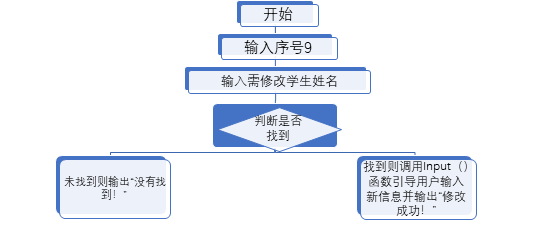（11）	清空学生信息：调用Studentmassage类的析构函数，将链表从头至尾挨个删除即可；
函数实现代码：
Studentmassage::~Studentmassage()
{
Save();
Student *temp;
//	cout << head->Next->CPP << "\n";
{
//		cout << temp->CPP << "\t";
delete temp;
}
//	cout << endl << head->CPP;
system("pause");
}

四、	测试结果：略
五、  软件使用说明书：
本软件采用命令行对话方式进行操作，运行后会出现在界面上，按照菜单提示进行操作即可。
且该软件每个功能都能选择是否需要继续使用，而不需要退回菜单后在重新选择；
需特别注意本程序中男性表示为male，女性表示为fama。
六、 源代码：
#include<iostream>
#include<fstream>
#include<cstring>
#include<conio.h>
#include<string>
using namespace std;

//-------------------Student类-------------------//
class Student
{
public:
char name;
char sex;
char ID;
double CPP;			//c++得分
double MATH;		//数学得分
double ENGLISH;		//英语得分
double sum;			//所得总分
int rank;			//学生排名
//	double avcpp;		//c++平均成绩
//	double avmath;		//数学平均成绩
//	double aveng;		//英语平均成绩
//	double avsum;		//总分平均成绩
Student *Next;

void Input();				//Input( )函数输入学生信息及成绩
void Show1();				//Show( )函数显示学生信息(for Display1)
void Show2();				//Show( )函数显示学生信息(for Display2)
};
//---------------Student类函数实现---------------//
void Student::Input()
{
cout << "\t\t 请输入学生姓名： ";		cin >> name;
cout << "\t\t 请输入学生性别： ";		cin >> sex;
cout << "\t\t 请输入学生的学号： ";		cin >> ID;
cout << "\t\t 请输入c++课程成绩： ";	cin >> CPP;
cout << "\t\t 请输入数学课程成绩： ";	cin >> MATH;
cout << "\t\t 请输入英语课程成绩： ";	cin >> ENGLISH;
sum = CPP + MATH + ENGLISH;
}
{
in >> name >> sex >> ID >> CPP >> MATH >> ENGLISH >> sum >> rank;
}
void Student::Show1()
{
/*	bool flag = true;
if (flag)
{
cout << "姓名: \t\t" << "性别: \t\t" << "学号: \t\t"
<< "C++: \t" << "数学: \t" << "英语：\t"
<< " 总成绩: \t" << "\n\n";
flag = false;
}*/
cout << name << "\t\t" << sex << "\t\t"
<< ID << "\t" << CPP << "\t" << MATH << "\t"
<< ENGLISH << "\t" << sum << "\n\n";

}
void Student::Show2()
{
/*	bool flag = true;
if (flag)
{
cout << "姓名: \t\t" << "性别: \t\t" << "学号: \t\t"
<< " 总成绩: \t" << "排名: " << "\n\n";
flag = false;
}*/
cout << name << "\t\t" << sex << "\t\t"
<< ID << "\t  " << sum << "\t\t" << rank << "\n\n";
}
//-----------------------------------------------------------------------------------//

//-------------------Studentmessage类-------------------//
class Studentmassage
{
public:
Studentmassage();
~Studentmassage();
void Find();
void Save();
void ModifyItem();
void RemoveItem();
void Swap(Student *, Student *);
void Sort();
int ListCount();
void Average();
void Display1();
void Display2();
private:
ifstream in;
ofstream out;
Student *FindItem(char *name)			//通过姓名查找
{
for (Student *p = head; p->Next != end; p = p->Next)
if (!strcmp(p->Next->name, name))	return p;
return NULL;
}
Student *FindID(char *ID)				//通过学号查找
{
for (Student *p = head; p->Next != end; p = p->Next)
if (!strcmp(p->Next->ID, ID))	return p;
return NULL;
}
};

//-------------------------------Studentmessage类函数实现-------------------------------//
//-------------------菜单-------------------//
{
cout << "\t\t**********************************************************************************" << endl;
cout << "\t\t\t〒〒〒〒〒〒〒〒〒〒  ☆ 学生成绩管理系统 ☆  〒〒〒〒〒〒〒〒〒〒" << endl;
cout << "\t\t〒〒〒〒〒〒〒★★★★★         ★★★★★★★〒〒〒〒〒〒〒" << endl;
cout << "\t\t〒〒〒〒〒〒〒〒〒★  ☆          1.读入学生信息          ☆  ★〒〒〒〒〒〒〒〒〒" << endl;
cout << "\t\t〒〒〒〒〒〒〒〒〒★  ☆          2.保存学生信息          ☆  ★〒〒〒〒〒〒〒〒〒" << endl;
cout << "\t\t〒〒〒〒〒〒〒〒〒★  ☆          3.添加学生信息          ☆  ★〒〒〒〒〒〒〒〒〒" << endl;
cout << "\t\t〒〒〒〒〒〒〒〒〒★  ☆          4.显示学生成绩(不含排名)☆  ★〒〒〒〒〒〒〒〒〒" << endl;
cout << "\t\t〒〒〒〒〒〒〒〒〒★  ☆          5.显示学生成绩(不含排名)☆  ★〒〒〒〒〒〒〒〒〒" << endl;
//	cout << "\t\t〒〒〒〒〒〒〒〒〒★  ☆          6.排序统计成绩          ☆  ★〒〒〒〒〒〒〒〒〒" << endl;
cout << "\t\t〒〒〒〒〒〒〒〒〒★  ☆          6.查看平均成绩          ☆  ★〒〒〒〒〒〒〒〒〒" << endl;
cout << "\t\t〒〒〒〒〒〒〒〒〒★  ☆          7.查找学生成绩          ☆  ★〒〒〒〒〒〒〒〒〒" << endl;
cout << "\t\t〒〒〒〒〒〒〒〒〒★  ☆          8.删除学生成绩          ☆  ★〒〒〒〒〒〒〒〒〒" << endl;
cout << "\t\t〒〒〒〒〒〒〒〒〒★  ☆          9.修改学生信息          ☆  ★〒〒〒〒〒〒〒〒〒" << endl;
cout << "\t\t〒〒〒〒〒〒〒〒〒★  ☆          10.清空学生信息         ☆  ★〒〒〒〒〒〒〒〒〒" << endl;
cout << "\t\t〒〒〒〒〒〒〒〒〒★  ☆          0.安全退出系统          ☆  ★〒〒〒〒〒〒〒〒〒" << endl;
cout << "\t\t\t\t系统中规定male为男性，fama为女性，请按该格式进行输入！！！" << endl;
cout << "\t\t**********************************************************************************" << endl;
cout << "\t\t\n\t\t  →请选择相应的数字进行操作: ";
}

//-------------------1.Studentmessage构造函数-------------------//
Studentmassage::Studentmassage()		//构造函数
{
in.open("sort.txt");
if (!in)	cout << "这是一个新系统，无学生信息，请先输入。" << endl;
else
{
while (!in.eof())
{
if (end->name == '\0')	break;
end->Next = new Student;
end = end->Next;
}
in.close();
cout << "\t\t 读取学生信息成功！" << endl;
}
}

//-------------------2.保存信息至文件中-------------------//
void Studentmassage::Save()
{
out.open("sort.txt");
for (Student *p = head->Next; p != end; p = p->Next)
{
out << p->name << "\t" << p->ID << "\t" << p->CPP << "\t" << p->MATH << "\t" << p->ENGLISH << "\t" << p->sum << p->rank << "\t" << endl;
}
out.close();
}

//-------------------3.添加新的学生信息-------------------//
{
end->Input();
//	scpp += end->CPP;
//	smath += end->MATH;
//	seng += end->ENGLISH;
end->Next = new Student;
end = end->Next;
cout << "添加成功！" << endl;
system("pause");
//	cout << "请输入任意字符！继续......";
//	getchar();
}

//-------------------4.输出信息-------------------//
void Studentmassage::Display1()
{
cout << "姓名: \t\t" << "性别: \t\t" << "学号: \t\t"
<< "C++: \t" << "数学: \t" << "英语：\t"
<< " 总成绩: \t" << "\n\n";
for (Student*p = head->Next; p != end; p = p->Next)
p->Show1();
system("pause");
//	cout << "输入任意字符！继续······";
//	getchar();
}

//-------------------5.输出只有总成绩和排名的信息-------------------//
void Studentmassage::Display2()
{
Sort();
cout << "姓名: \t\t" << "性别: \t\t" << "学号: \t\t"
<< " 总成绩: \t" << "排名: " << "\n\n";
for (Student*p = head->Next; p != end; p = p->Next)
p->Show2();
system("pause");
//	cout << "输入任意字符！继续······";
//	getchar();
}

//-------------------6.计算各科平均成绩-------------------//
void Studentmassage::Average()
{
double scpp = 0.0, smath = 0.0, seng = 0.0, ssum = 0.0;
int c = 0, m = 0, e = 0;	//记录各科不及格人数
Student *p;
for (p = head->Next; p->Next != end; p = p->Next)
{
scpp += p->CPP; smath += p->MATH; seng += p->ENGLISH; ssum += p->sum;
if (p->CPP < 60) c++;
if (p->MATH < 60) m++;
if (p->ENGLISH < 60) e++;
//		cout << p->CPP << endl;
}
scpp += p->CPP; smath += p->MATH; seng += p->ENGLISH; ssum += p->sum;
int num = Studentmassage::ListCount();
//	cout << num << endl;
double avcpp = scpp / num;			//c++平均成绩
double avmath = smath / num;		//数学平均成绩
double aveng = seng / num;			//英语平均成绩
double avsum = ssum / num;			//总分平均成绩
double procpp = (num - c) / num;	//c++及格率
double promath = (num - m) / num;			//数学及格率
double proeng = (num - e) / num;			//英语及格率
//	cout << scpp << "\t" << smath << "\t" << seng << "\t" << ssum << "\n";
cout << "\n\t\t*********************************\n";
cout << "\t\t※ 0.输出各项平均成绩\n";
cout << "\t\t※ 1.输出c++平均成绩 \n\t\t※ 2.输出数学平均成绩 \n";
cout << "\t\t※ 3.输出英语平均成绩 \n\t\t※ 4.输出总平均成绩 \n";
cout << "\t\t※ 5.输出各科及格率";
cout << "\n\t\t*********************************\n";
char flag = 'n';
do
{
cout << "\t\t →请选择相应的数字进行操作:： ";
int x; cin >> x;
switch (x)
{
case 0: {
cout << "\t\tc++平均成绩\t" << "数学平均成绩\t" << "英语平均成绩\t" << "总平均成绩\t\n";
cout << "\t\t" << avcpp << "\t\t" << avmath << "\t\t" << aveng << "\t\t" << avsum << "\n\n"; break;
}
case 1:cout << "\t\tc++平均成绩 : " << avcpp << "\n\n"; break;
case 2:cout << "\t\t数学平均成绩 : " << avmath << "\n\n"; break;
case 3:cout << "\t\t英语平均成绩 : " << aveng << "\n\n"; break;
case 4:cout << "\t\t总平均成绩 : " << avsum << "\n\n"; break;
case 5:
{
cout << "\t\tc++及格率\t" << "数学及格率\t" << "英语及格率\t" << "\n\n";
cout << "\t\t" << procpp * 100 << "%" << "\t\t" << promath * 100 << "%"
<< "\t\t" << proeng * 100 << "%" << "\t\t" << "\n\n"; break;
}
}
cout << "\t\t请问是否要继续查询？(y/n) : ";
cin >> flag;
} while (flag == 'y');
system("pause");
}

//-------------------7.查找函数-------------------//
void Studentmassage::Find()
{
char c = 'n';
do
{
char name, ID;
Student *p = NULL;
cout << "\n\t\t*********************************\n";
cout << "\t\t※ 1.按学生的姓名查找 \n\t\t※ 2.按学生学号查找 ";
cout << "\n\t\t*********************************\n 请选择： ";
int x;
cin >> x;
switch (x)
{
case 1:
{
cout << "\t\t 请输入要查找学生姓名：  ";
cin >> name;
if (p = FindItem(name))
{
p->Next->Show1();
//			cout << "输入任意字符！继续······ \n";
//			getchar();
}
else
{
cout << "\t\t 没有找到相匹配的学生！  " << "\n\n";
//			cout << "请输入任意字符！继续······";
//			getchar();
}
} break;
case 2:
{
cout << "\t\t 请输入要查找的学生的学号： "; cin >> ID;
if (p = FindID(ID))
{
p->Next->Show1();
//			cout << " 输入任意字符！继续…… ";
//			getchar();
}
else
{
cout << "\t\t 没有找到相匹配的学生！ " << '\n' << endl;
//			cout << " 输入任意字符！继续…… ";
//			getchar();
}
} break;
}
cout << "\t\t请问是否要继续查找？(y/n) : ";
cin >> c;
} while (c == 'y');
system("pause");
}

//-------------------8.删除函数-------------------//
void Studentmassage::RemoveItem()
{
char c = 'n';
do
{
char name;
Student *p = NULL, *temp = NULL;
cout << "\t\t 请输入要删除学生的姓名 ：" << endl; cin >> name;
if (p = FindItem(name))
{
temp = p->Next;
p->Next = p->Next->Next;
delete temp;
cout << "\t\t 删除成功！" << endl;
//		cout << "输入任意字符！继续······";
//		getchar();
}
else
{
cout << "\t\t 没有找到！" << endl;
//		cout << "输入任意字符！继续······";
//		getchar();
}
cout << "\t\t请问是否要继续删除？(y/n) : ";
cin >> c;
} while (c == 'y');
system("pause");
}

//-------------------9.修改函数-------------------//
void Studentmassage::ModifyItem()
{
char c = 'n';
do
{
char name;
Student *p = NULL;
cout << "\t\t 请输入需要修改人的姓名 ："; cin >> name;
if (p = FindItem(name))
{
cout << "\t\t 已找到该学生信息，请按步骤输入新的信息！" << endl;
p->Next->Input();
cout << "修改成功！ " << endl;

//		cout << "请输入任意字符！继续······";
//		getchar();
}
else
{
cout << "\t\t 没有找到！" << endl;
//		cout << "请输入任意字符！继续······";
//		getchar();
}
cout << "\t\t请问是否要继续修改？(y/n) : ";
cin >> c;
} while (c == 'y');

system("pause");
}

//-------------------10.Studentmessage析构函数-------------------//
Studentmassage::~Studentmassage()
{
Save();
Student *temp;
//	cout << head->Next->CPP << "\n";
{
//		cout << temp->CPP << "\t";
delete temp;
}
//	cout << endl << head->CPP;
delete end;
system("pause");
}

//-------------------记录当前链表总数并返回一个整数-------------------//
int Studentmassage::ListCount()
{
int n = 0;
for (Student *p = head->Next; p != end; p = p->Next)	n++;
return n;
}

//-------------------交换Student类的两个变量-------------------//
void Studentmassage::Swap(Student *p1, Student *p2)
{
Student *temp = new Student;
strcpy(temp->name, p1->name);
strcpy(temp->sex, p1->sex);
strcpy(temp->ID, p1->ID);
temp->CPP = p1->CPP;
temp->MATH = p1->MATH;
temp->ENGLISH = p1->ENGLISH;
temp->sum = p1->sum;

strcpy(p1->name, p2->name);
strcpy(p1->sex, p2->sex);
strcpy(p1->ID, p2->ID);
p1->CPP = p2->CPP;
p1->MATH = p2->MATH;
p1->ENGLISH = p2->ENGLISH;
p1->sum = p2->sum;

strcpy(p2->name, temp->name);
strcpy(p2->sex, temp->sex);
strcpy(p2->ID, temp->ID);
p2->CPP = temp->CPP;
p2->MATH = temp->MATH;
p2->ENGLISH = temp->ENGLISH;
p2->sum = temp->sum;
}

//-------------------对当前链表进行排序-------------------//
void Studentmassage::Sort()
{
cout << "Sorting..." << endl;
Student *p = NULL, *p1 = NULL, *k = NULL;
int n = Studentmassage::ListCount();
if (n < 2)	return;
for (p = head->Next; p != end; p = p->Next)
for (k = p->Next; k != end; k = k->Next)
{
if (p->sum < k->sum)	Studentmassage::Swap(p, k);
}
int i = 1;
for (p = head->Next; p != end; p = p->Next)
{
p->rank = i++;
}
cout << "排序完成！" << endl;
system("pause");
//	getchar();
return;
}
//-----------------------------------------------------------------------------------//

int main()
{
int x, i = 0;
bool quit = false;
cout << "\t\t §§§§§§§§§§§§§§§§§§§§§§§§§§ \n" << endl;
system("pause");
//	cout << "\t\t按任意键开始······";
//	getchar();
while (!quit)
{
cin >> x;
switch (x)
{
case 0:exit(0); break;
}
}
return 0;
}

//计算平均成绩有问题(解决)
//序号选择应添加筛错选项
//Student类应在添加性别选项
//析构函数第一个数据无法删除
//清空链表后Student类Show函数出现异常



展开全文c++
• Python实现的学生信息管理系统引言功能需求2.1 功能菜单的实现2.2 录入学生信息2.3 删除学生信息2.4 修改学生信息2.5 查找学生信息2.6 查找学生总人数2.7 查看学生数据2.8 排序学生数据总结合理的创建标题，有助于...
Python实现的学生信息管理系统引言功能需求2.1 功能菜单的实现2.2 录入学生信息2.3 删除学生信息2.4 修改学生信息2.5 查找学生信息2.6 查找学生总人数2.7 查看学生数据2.8 排序学生数据总结
引言
学完了Python的基础知识，那必然要通过实践来巩固一下，通过软件开发，进一步掌握并加强软件工程的方法和技术，提高自己的软件开发实际能力，提高自己的创造能力、工程设计能力、解决问题能力、综合分析能力以及锻炼自己创造性的思维。为此，我们制作了一个非常简单的学生信息管理系统
功能需求
该系统主要实现以下功能：
      1.录入学生信息
2.查找学生信息
3.删除学生信息
4.修改学生信息
5.排序
6.统计学生总人数
7.查看学生信息

2.1 功能菜单的实现
在系统运行过程中，始终为用户展示功能菜单，以便用户操作。
def menu():
print("================================学生信息管理系统====================================")
print('------------------------------------功能菜单---------------------------------------')
print('\t\t\t\t\t\t1.录入学生信息')
print('\t\t\t\t\t\t2.查找学生信息')
print('\t\t\t\t\t\t3.删除学生信息')
print('\t\t\t\t\t\t4.修改学生信息')
print('\t\t\t\t\t\t5.排序')
print('\t\t\t\t\t\t6.统计学生总人数')
print('\t\t\t\t\t\t7.查看学生信息')
print('\t\t\t\t\t\t0.退出系统')
print('---------------------------------------------------------------------------------')

菜单函数实现完成后，如何选择我们的功能呢?下面就看一下我们的控制流程
def main():
while True:
try:
choice = int(input('请选择'))
if choice in range(8):
if choice == 0:
print('感谢您的使用!')
break
elif choice == 1:
insert()
elif choice == 2:
search()
elif choice == 3:
delete()
elif choice == 4:
update()
elif choice == 5:
sort()
elif choice == 6:
total()
elif choice == 7:
show()
else:
print("您的输入有误,请重新输入")

except:
print("您的输入有误,请重新输入")

if __name__ == '__main__':
main()


是不是很简单呢？
下面就开始实现本系统的第一个功能吧
2.2 录入学生信息
针对我们输入的数据，我们会把它存储在我们的磁盘上，因此创建一个save()函数来完成这个操作
先说一下我们的这个save(lst)函数，需传入一个list列表类型的参数lst，因为我们的每一条学生信息都是作为字典类型存储在列表中的。
声明：这里的fileName是我们提前定义好的文件名称
fileName = 'student.txt'

这样再来看我们的save函数，是不是顺眼了许多呢？
def save(lst):
# 读取磁盘学生列表的内容
try:
file = open(fileName, 'a', encoding='utf-8')
except:
# 文件不存在,以写的方式创建文件
file = open(fileName, 'w', encoding='utf-8')
for student in lst:
# 将每行的数据转化为字符串
file.write(str(student) + '\n')
file.close()
print("学生信息录入完毕!!!")


有了save(lst)函数，下面就可以写我们的insert()函数来录入学生信息了
需注意：学号、姓名不能为空，学生的成绩必须为整数类型，否则会抛出异常重新输入。
def insert():
studentList = []
while True:
stuNo = input("请输入学生的ID:")
if not stuNo:
print("学生的ID不能为空,请重新输入")
continue
stuName = input("请输入学生的姓名:")
if not stuName:
print("学生的姓名不能为空,请重新输入")
continue
while True:
try:
java = int(input("请输入学生的Java成绩:"))
python = int(input("请输入学生的Python成绩:"))
cc = int(input("请输入学生的C++成绩:"))
except:
print("学生的成绩必须为整数,请重新输入")
continue
else:
break
# 将录入的学生信息保存在字典中
student = {'id': stuNo, 'name': stuName, 'java': java, 'python': python, 'cc': cc}
# 将学生信息添加在列表中
studentList.append(student)
continue
else:
break
# 将录入的信息存入磁盘中
save(studentList)

当学生信息都存储在studentList列表中后,在代码段的最后调用我们创建好的save(lst)函数将学生信息存储在磁盘中即可。
录入数据后查看一下我的磁盘文件student.txt2.3 删除学生信息
若要删除学生信息，那么肯定少不了读取磁盘文件,这就需要我们引入os模块来判断磁盘文件是否存在
引入os模块的命令：import os
def delete():
while True:
id = input("请输入你要删除的学生ID")
# 判断磁盘文件是否存在
if id:
if os.path.exists(fileName):
# 从磁盘文件中读取文件
with open(fileName, 'r', encoding='utf-8') as file:
else:
print("磁盘中无任何学生信息")
break
flag = False  # 判断是否删除学生记录
if studentList:
with open(fileName, 'w', encoding='utf-8') as file:
for student in studentList:
#将字符串类型的student转化为字典类型
student = eval(student)
if student['id'] != id:
file.write(str(student) + '\n')
else:
flag = True
if flag:
print(f"学号为{id}的学生信息删除成功")
break
else:
print(f"没有找到学号为{id}的学生")
show()
else:
print("输入的学生ID不能为空,请重新输入")
continue
continue
else:
break


在这里我们使用了一个with语句，使用with语句的好处就在于不用我们手动关闭文件流。
引入一个博主的话就是：with 语句适用于对资源进行访问的场合，确保不管使用过程中是否发生异常都会执行必要的“清理”操作，释放资源，比如文件使用后自动关闭、线程中锁的自动获取和释放等。
如果仍然对with语句留有疑惑，那么可以去参考这篇博客：https://www.cnblogs.com/xiaxiaoxu/p/9747551.html，相信你会有更加深刻的理解！
2.4 修改学生信息
实现这个功能的一个基本思想就是：
从磁盘读取文件，依次遍历数据，如果数据项的id与输入的要修改学生的id相同，则修改他的信息并写入磁盘文件，如果id不同，则将原信息写入文件。
def update():
show()
while True:
# 先从磁盘读取学生信息
if os.path.exists(fileName):
with open(fileName, 'r', encoding='utf-8') as file:
else:
print("磁盘中不存在学生信息\n")
break
id = input("请输入要修改的学生ID")
flag = False  # 判断是否存在学号为id的学生信息
if id:
with open(fileName, 'w', encoding='utf-8') as file:
for student in studentList:
# 将学生信息转化为字典类型
student = eval(student)
if student['id'] == id:
flag = True
print(f"找到学号为{id}的学生了,可以进行修改")
while True:
try:
student['name'] = input("请输入学生的姓名")
if not student['name']:
print("学生的姓名不能为空,请重新输入")
continue
student['java'] = int(input("请输入学生的Java成绩:"))
student['python'] = int(input("请输入学生的Python成绩:"))
student['cc'] = int(input("请输入学生的C++成绩:"))
break
except:
print("你的输入有误,请重新输入")
file.write(str(student) + '\n')

else:
print("学生的id不能为空,请重新输入")
continue
if not flag:
print(f"不存在学号为{id}的学生")
else:
print("学生信息修改完毕!!!")
continue
else:
break


2.5 查找学生信息
这里我们提供两种查找方法:
		1.根据学生ID查找
2.根据学生姓名查找

当查找完数据后，我们需要对数据进行展示，如果我们直接输出，那么数据必然杂乱无章，影响用户体验，因此我们创建一个show_search(lst)方法为输出的数据加上一定的格式，使输出的数据简洁美观。
# 按照一定格式列出学生信息
def show_search(studentQuery):
if len(studentQuery) == 0:
print("没有查找到学生信息,无数据显示!!!")
# 定义标题显示格式
title_format = '{:^6}\t{:^12}\t{:^8}\t{:^10}\t{:^10}\t{:^8}\t'
print(title_format.format('ID', '姓名', 'Java成绩', 'Python成绩', 'C++成绩', '总成绩'))
# 定义数据显示格式
data_format = '{:^6}\t{:^12}\t{:^8}\t{:^10}\t{:^10}\t{:^8}\t'
for student in studentQuery:
print(data_format.format(student.get('id'),
student.get('name'),
student.get('java'),
student.get('python'),
student.get('cc'),
int(student.get('java')) + int(student.get('python')) + int(student.get('cc'))))


下面给出我们的查找函数search()的相关操作:
def search():
studentQuery = []
while True:
id = ''
name = ''
if os.path.exists(fileName):
mode = int(input("按ID查找输入1,按姓名查找请按2:"))
if mode == 1:
id = input("请输入要查找学生的id")
elif mode == 2:
name = input("请输入要查找学生的姓名")
else:
print("输入格式错误,请重新输入")
search()
return
with open(fileName, 'r', encoding='utf-8') as file:
for student in studentList:
student = eval(student)
if id:
if student['id'] == id:
studentQuery.append(student)
if name:
if student['name'] == name:
studentQuery.append(student)
show_search(studentQuery)
# 清空查找列表
studentQuery.clear()
continue
else:
break
else:
print("磁盘上不存在学生信息文件")
return


在上面的search()函数中，每当我们调用show_search(lst)函数后，需要清空学生列表数据，这是为了避免再次查找学生信息时，将上一次查找的信息也带了进去，导致程序错乱。
2.6 查找学生总人数
该功能比较简单，只需读取文件，然后遍历数据即可，不再过多简述，直接上代码。
def total():
if os.path.exists(fileName):
with  open(fileName, 'r', encoding='utf-8') as file:
if studentList:
print("共有{0}名学生\n".format(len(studentList)))
else:
print("还没有录入学生信息\n")

else:
print("磁盘中没有学生信息文件!!!\n")

2.7 查看学生数据
因为我们已经有了show_search(lst)格式化输出函数，因此我们只要读取文件拿到数据列表，再调用show_search(lst)函数即可。
def show():
studentQuery = []
if os.path.exists(fileName):
with  open(fileName, 'r', encoding='utf-8') as file:
for student in studentList:
student = eval(student)
studentQuery.append(student)
show_search(studentQuery)
# 清空列表
studentQuery.clear()
else:
print("磁盘中没有学生信息文件!!!\n")

哈哈，历经艰辛，终于快结束了！！！
别急，坚持一下，做完这个排序函数，才算大功告成。
2.8 排序学生数据
提到排序，那么必然有【升序】【降序】两个选择
其次，要根据什么进行排序吗？也就是我们的排序依据是什么呢？
这里我们给出了四个选择
	1. 根据Java成绩排序
2. 根据Python成绩排序
3. 根据C++成绩排序
4. 根据总成绩排序

这里我用到了Python中的一个内置函数sort() ，sort方法还有两个可选参数：key和reverse
key：排序所依据的关键词，在这里我们使用的是lamdba函数，是固定写法，不要写成别的单词，dict表示列表中的一个元素，在这里，表示一个元组，dict只是临时起的一个名字，你可以使用任意的名字；
对lamdba不理解的，参考这个链接：https://www.cnblogs.com/zle1992/p/6271105.html
reverse：reverse指定排序方式，需要提供一个布尔值：True为降序，False为升序
def sort():
show()
studentList = []
if os.path.exists(fileName):
with open(fileName, 'r', encoding='utf-8') as file:
for student in students:
student = eval(student)
studentList.append(student)
ascOrDesc = int(input("请选择(0.升序,1.降序)"))
if ascOrDesc == 0:
ascOrDescBool = False
elif ascOrDesc == 1:
ascOrDescBool = True
else:
print("数据输入不合法,请重新输入")
sort()
return
while True:
mode = int(input('请选择排序方式(1.按Java成绩排序 2.按Python成绩排序 3.按C++成绩排序 0.按总成绩排序\n)'))
if mode == 0:
studentList.sort(key=lambda dict:int(dict['java'])+int(dict['python'])+int(dict['cc']),reverse=ascOrDescBool)
elif mode == 1:
studentList.sort(key=lambda dict: int(dict['java']) ,reverse=ascOrDescBool)
elif mode == 2:
studentList.sort(key=lambda dict: int(dict['python']), reverse=ascOrDescBool)
elif mode == 3:
studentList.sort(key=lambda dict: int(dict['cc']), reverse=ascOrDescBool)
else:
print("输入数据不合法,请重新输入")
continue
show_search(studentList)
break
sort()
else:
print("磁盘中不存在学生信息\n")

总结
到这里本系统的制作就结束了，这也是本人所写的第一篇博客文章，希望可以帮到那些初学Python的小伙伴们，如果上述代码有任何问题或者疑惑，欢迎大家评论指出，日后一定改进。
今后也会陆续向大家分享自己所学的知识，希望大家关注，不喜勿喷。


展开全文• 文档标识：201091601学生信息管理系统软件测试编 写 李宝刚校 对小组成员 李宝刚孔维一李宇杰二OO年目录1.引言11.1.目的11.2.背景11.3.范围11.4.定义11.5.参考资料12.测试内容13.测试规则23.1.进入准则23.2.暂停/...
文档标识：201091601学生信息管理系统软件测试编 写         李宝刚校  对小组成员         李宝刚孔维一李宇杰二OO年目录1.引言11.1.目的11.2.背景11.3.范围11.4.定义11.5.参考资料12.测试内容13.测试规则23.1.进入准则23.2.暂停/退出准则23.3.测试方法23.4.测试手段33.5.测试要点33.6.测试工具44.测试环境44.1.硬件环境44.2.软件环境44.3.安全性环境要求45.项目任务45.1.测试规划45.2.测试设计45.3.测试执行准备55.4.测试执行65.5.测试总结76.实施计划86.1.工作量估计86.2.人员需求及安排86.3.进度安排86.4.其他资源需求及安排96.5.可交付工件97.风险管理9引言1.1.目的随着学校规模不断扩大,学生数量急剧增加，有关学生的信息量也成倍增长，面对庞大的信息量需要有学生管理系统来提高学生管理工作的效率。本系统主要用于学校学生信息管理，总体任务是实现学生信息关系的系统化、规范化、自动化，其主要任务是用计算机对学生各种信息进行日常管理，如查询、修改、增加、删除，另外还考虑到学生选课，针对了这些要求设计了学生信息管理系统。1.2.背景在高校，计算机应用的非常普遍，在这种实用的学生信息管理系统可以使局面得到改观。学生信息管理系统主要提供了方便高校的管理功能以及网上信息的查阅平台，学生可以通过该系统查询相关信息，管理员可以管理信息，本系统主要功能有：学生管理功能：为了方便学生信息的增加、删除、修改、查询。课程管理功能：管理员可以通过填写表格的形式修改课程等相关信息。成绩管理功能：管理员可以通过数据库中的学生成绩信息进行增加、修改。班级管理功能：管理员可以通过此功能对班级信息进行增加、删除、修改、查询。用户管理功能：可以增加、删除、修改、查看该程序的用户登录，超级管理员可以设置用户的权限。1.3.范围本学生信息管理系统主要应用在各个学校为了方便管理学生信息而成。主要设计人员由在校学生以及老师组成。测试风险有可能软件应用过程中出现一些错误或者故障。时间进度：2010-7 ----1.4.定义学生管理系统   信息管理   数据库  软件测试1.5.参考资料资料名称作者日期出版单位1《软件测试自动化》邓波 黄丽娟 曹青春机械工业出版社2《有效软件测试》Elfriede Dustin     1990清华大学出版社    3《软件测试周予滨 姚静列出编写本计划时需查阅的Intenet上杂志、专业著作、技术标准。查阅内容网点地址简介软件测试/16822&ref=ps软件测试入门软件测试教程/1117943.htm教你如何学会软件测试测试内容下表列出了学生信息管理系统项目的测试需求，并对其进行了优先级定义：子系统名称模块名称测试点优先级说明学生信息管理系统学生信息管理模块数据输入是否正常H实现增、删、改、查数据输入是否正常H实现增、删、改、查数据输入是否正常H实现增、删、改、查 查询系统模块数据连接是否正常H连接正常数据库管理系统数据连接是否正常H连接正常     用户管理系统 登入是否正常H连接正常连接是否正常H连接正常测试规则3.1.进入准则一个软件要想进去准备阶段首先要达到一下三个阶段：A．需求工件已经被基线化B．详细设计工件已经被基线化C．构架工件已经被基线化2.1 集成测试退出标准 1) 集成测试用例设计已经通过评审 2) 所有源代码和可执行代码已经建立受控基线，纳入[url=javascript:;]配置管理[/url]受控库，不经过审批不能随意更改 3) 按照集成构件计划及增量集成策略完成了整个系统的集成测试 4) 达到了测试计划中关于集成测试所规定的覆盖率的要求 5) 集成工作版本满足设计定义的各项功能、性能要求 6) 在集成测试中发现的错误已经得到修改，各级缺陷修复率达到标准 7) A、B类BUG不能存在 8) C、D类BUG允许存在,但不能超过单元测试总BUG的5
展开全文• C语言学生信息管理系统设计实验报告 中南民族大学中南民族大学 软件课程设计报告软件课程设计报告 电信电信 学院学院 1010 级级光信光信专业专业 题目题目学生信息管理系统学生信息管理系统 姓名姓名 张宇(组长)张宇...C语言学生信息管理系统设计实验报告 中南民族大学中南民族大学 软件课程设计报告软件课程设计报告 电信电信 学院学院 1010 级级光信光信专业专业 题目题目学生信息管理系统学生信息管理系统 姓名姓名 张宇(组长)张宇(组长) 钱程钱程 刘永亮刘永亮 马玉帅马玉帅 岳映强岳映强 章成章成 陈耀明陈耀明 徐川鹤徐川鹤 学号学号 1007406810074068 1007406910074069 1007407010074070 1007407110074071 1007407210074072 1007407310074073 1007406710074067 1007406010074060 指导教师指导教师谢茂涛谢茂涛 2011 年年06 月月11 日日 2 1.1.引言引言 本课程设计的目的就是要通过一次集中的强化训练，使学生能及时巩固已学 的知识，补充未学的但又必要的内容。进行课程设计目的在于加深对程序设计基 础中基础理论和基本知识的理解，促进理论与实践的结合，进一步提高程序设计 的能力。具体目的如下： 1．使学生更深入地理解和掌握该课程中的有关基本概念，程序设计思想和 方法。 2．培养学生综合运用所学知识独立完成课题的能力。 3．培养学生勇于探索、严谨推理、实事求是、有错必改，用实践来检验理 论，全方位考虑问题等科学技术人员应具有的素质。 4．提高学生对工作认真负责、一丝不苟，对同学团结友爱，协作攻关的基 本素质。 5．培养学生从资料文献、科学实验中获得知识的能力，提高学生从别人经 验中找到解决问题的新途径的悟性，初步培养工程意识和创新能力。 6．对学生掌握知识的深度、运用理论去处理问题的能力、实验能力、课程 设计能力、书面及口头表达能力进行考核。 2.2.设计题目及要求设计题目及要求 【1】设计题目 ：学生信息管理系统 【2】要求 实现如下功能： (1)建立学生信息的结构体 (2)学生信息的初始化(可以从文件获取) (3)学生信息的添加、修改、删除、查找 (4)学生信息的输出 (5)对学生信息按学号、姓名查找 (6)将学生信息保存为文件 综合应用数组、指针、结构体、文件等，对该设计题目进行设计和实现。课 程设计完成后，提交课程设计报告。 3.3.设计算法分析设计算法分析 3.1 模块调用层次图 【1】变量描述 定义形式/名称含义/作用 数组char name定义姓名数组 结构体struct student work声明一个结构数组 全局变量int =0;float ave,sum; FILE *fp; 全局变量标记当前 录入学生个数 文件work_list.txt保存学生信息 3 函数baicun()、type_in()、 del()、change()、add ()、scan()search() 保存 录入 删除 修改 查找 插入 显示学生信息、查 找 4.4.设计平台设计平台 有 Visual C 语言编写，语言简单、紧凑，使用方便、灵活。运算符丰富，数据 类型丰富，具有结构化的控制语句，语法限制不太严格，程序设计自由度大。C 语言允许直接访问物理地址，能进行行位操作，能实现汇编语言的大部功能。生 产目标代码质量高，程序执行效率高，用 C 语言编写的程序可移植性好。 5.5.设计思想设计思想 采用了系统模块化：(1)自顶向下；(2)逐步细化；(3)模块化设计；(4) 结构化编码。用这种方法便于验证算法的正确性，在向下展开之前应仔细检查本 层设计是否正确，只有上一层正确才能向下细化。本程序分为六大模块：浏览模 块、增加模块、录入模块、修改模块、查询模块、退出。其中录入模块包括录入、 导出、保存。查询模块包括学号查询和姓名查询。浏览函数由岳映强完成，增添 模块由马玉帅完成，录入模块由章成完成，修改模块由陈耀明和徐川鹤完成，查 询模块由刘永亮完成，退出模块由钱程完成。 6.6.设计框图设计框图 Main 函数 信 息 的 浏 览 信 息 的 增 加 信 息 的 录 入 信 息 的 修 改 信 息 的 查 询 退 出 系 统 按 学 号 查 询 按 姓 名 查 询 信 息 保 存 信 息 录 入 信 息 导 出 7.各模块功能简介各模块功能简介 信息浏览模块：用导入函数调入信息，用 for 循环依次输出学生信息。 4 信息的增添模块：先确定增添人数，用 for 循环输入指针数组数据，调用保存函 数进行保存。 信息的录入模块：确定人数，for 循环依次输入信息，调用保存函数保存。 信息的修改模块：用 if(strcmp(work[i].name,name)==0) 先找出要修改的学生信息，用 switch 确定修改那一项，输入修改的数据进行保 存。 信息的查询模块：用 if(strcmp(work[i].name,name)==0)查找学生姓名，用 if(num1==work[i].num)对学号查找。 退出：用 goto 语句指向固定行。 8.主要函数流程图主要函数流程图 修改函数 开始 输入姓名 用 strcmp 查找 是否找到 输入选项 修 改 姓 名 修 改 学 号 修 改 年 龄 修 改 性 别 修 改 地 址 修 改 电 话 修 改 生 日 修 改 邮 箱 是否继续 Case1－8 N N Y Y 结束 5 查询函数 开始 输入查询姓名 用 strcmp 比较 是否找到 输出信息 结束 Y N 增加函数 开始 输入人数 输入信息 调用保存函数 是否继续 结束 Y N 删除函数 6 开始 输入姓名 用 strcmp 比较 是否找到 输出信 是否删除 指针前移 结束 是否继续 Y N Y N N Y 9.9.程序调试及分析程序调试及分析 经调试大部分功能以实现，只有学号查询出错，可能原因：指针指向出错，调用 后带回空指针。电话的数据类型不能输出全部电话位数，因为定义的长整型最大 位数小于电话位数。 1010、结束语、结束语 7 通过本次实验对 C 语言有了初步了解，但如果想要进一步了解，还应加强练习， 组员之间的配合在设计过程中是很重要的。 参考资料：参考资料：C 程序设计C 语言程序基础
展开全文• 学生信息管理系统是一个教育单位不可缺少的部分,它的内容对于学校的决策者和管理者来说都至关重要,所以学生信息管理系统应该能够为用户提供充足的信息和快捷的查询手段 1.2在国内外发展概况及存在问题 一直以来人们...
• Java 程序设计课程设计 目录 摘要 - 2 - 一引言 - 3 - 一问题提出 - 3 - 二需求与系统分析 - 3 - 1 需求分析 - 3 - 2 系统分析 - 3 - 二程序的主要功能 - 4 - 一录入学生信息 - 4 - 二查询学生信息 - 4 - 三修改学生...
• 学生信息管理系统需求分析报告 包括 引言 需求规定 数据描述 数据字典 输入输出需求
• 4.1.6 学生信息管理模块 36 4.1.7 课程信息管理模块 49 4.1.8 成绩信息管理模块 54 4.1.9 年份信息管理模块 58 4.2 系统工作流程（时序图） 61 第五章 系统测试 62 结束语 63 致谢 64 主要参考文献 65 附录（附图） ...
• 引言：Java实现学生信息管理系统，主要有一种是GUI图形界面实现，另一种是控制台输出，还有java web实现的，（基于Maven)甚至用SSM框架实现，springboot等都是可以的，但底层语言都是用的Java 语言，如果有需要类似...
• 随着学校的规模逐渐扩大，学校的招生越来越多，新进来的学生和以前的学生 档案管理规模越来越大，学生信息管理存在不安全，不完整，不真实的隐患。学校的管理者需要对学生负责，要高效的，安全的，真实的获取学生...
• 学生信息管理系统》论文 摘要 学生信息管理系统是一个教育单位不可缺少的部分，它的内容对于学校的决策者和管理者来说都至关重要。本文主要介绍了学生信息管理系统的开发，包括了项目开发目的、系统结构、实验代码...python
• 8.学生管理模块 45 9. 成绩管理模块 46 10. 课程管理模块 48 （二） 测试 49 1. 模块测试 49 2. 子系统测试 91 3. 系统测试 102 4. 验收测试 103 六、总结与展望 123 （一）总结 123 （二）展望 124 包括源码+...
• ## 学生作业信息管理系统

万次阅读 多人点赞 2018-06-23 00:36:18
其次，答题信息管理系统可以大大提高老师与学生之间的在家庭作业和考核之间交流的效率，同时由于对题目以及学生成绩进行了数字化，可以对学生成绩及老师教学效果进行大数据分析。     最...JAVA easyui 数据库 jsp
• 基于Python的学生信息管理系统周庆潘学文（湖南科技学院电子与信息工程学院，湖南永州425199）【摘要】针对传统的人工管理学生信息效率低下、安全性差的问题，利用计算机和Python语言进行学生信息管理。系统框架使用...
• 基于Web的学生信息管理信息系统。 面对信息时代的高速发展和在信息化发展过程中出现的各种情况，该学生信息信息管理系统在实施后可以达到以下目标：（1）学生用户：包括修改密码、重新登录、查看个人基本信息、查看...java 数据库 mysql
• 成 绩 江 西 科 技 师 范 大 学 毕业设计论文 学生姓名 题目中文 基于 Java 的学生信息管理系统 Student Information Management System 外文 based on Java 院系 数学与计算机科学学院 专 业 计算机科学与技术 年 ...
• 成绩: 江西科技师范大学 毕业设计论文 学生姓名 题目中文 基于Java的学生信息管理系统 外文 Student Information Management System based on Java 院系 数学与计算机科学学院 专 业 计算机科学与技术 年 级 学号 ...
• 1.项目引言 1.1项目介绍 下学生入校后，我们需要管理这些学生，那么就需要我们对这些学生进行很多的 ... 这是一个学生信息管理系统，应用的是SSM(Spring + SpringMVC + Mybatis) 的项目，运用的知识： Sprin...
• 基于Python的学生信息管理系统周庆潘学文【摘要】【摘要】针对传统的人工管理学生信息效率低下、安全性差的问题，利用计算机和Python语言进行学生信息管理。系统框架使用Python语言中的Django框架，利用HTML技术进行...
• 总体任务是实现学生信息关系的系统化、规范化、自动化，其主要任务是用计算机对学生各种信息进行日常管理，如查询、修改、增加、删除，另外还考虑到学生选课，针对了这些要求设计了学生信息管理系统。 1.2 背景测试计划书
• 对于软件工程课程，所写的学生信息管理系统需求分析包含 第一章 引言 第二章 需求规定 第三章 图形描述系统第四章 系统特点 等章节组成需求分析
• 学生信息管理系统 目 录 TOC \o "1-3" \h \z \u 1. 论 文 摘 要 6 2.引言 7 3.系统开发环境 9 3.1 系统开发理论依据 9 3.2 系统开发环境 12 4.系统分析 14 4.1 系统目标 14 4.2 可行性分析 14 5系统结构 16 5.1平台...
• 一．引言 1.开发背景 在网络迅速发展的今天，教育事业的发展也逐步实行网络化，校园的管理也开始公开化和网络化，为了让同学们更好，... 开发一个学生信息管理系统,可以对学生的基本信息,成绩信息进行查询,修改等功能
• 数据库课程设计——学生宿舍信息管理系统 目录  1.设计目的... 2 2、任务与要求... 2 3、学生宿舍管理系统课程设计... 2 3.1 引言... 2 3.2需求分析... 2 3.2.1....
• 1．引言 1 1.1 项目背景 1 1.2 编写目的 1 1.3 软件定义 1 1.4 开发环境 2 2．需求分析 2 2.1 信息需求 2 2.2 处理需求 2 2.3 安全性与完整性要求 2 3．概念结构设计 4 4．逻辑结构设计 5 5．主要模块的设计以及代码...
• 我的第一个C++项目。粗糙的学生信息管理系统。用TXT文件来做数据持久化。C++
• 学生社团管理系统 1引言 1.1 项目来源 通过对各大高校实地的调查可知一般高校的学生社团信息管理主要是依据纸质和手工作业处理人工的对大量会员的基本资料进行档案式管理此种处理方式数据量大管理模式和方法滞后 ...
• 引言 一、定义学生类 二、编写学生接口 三、连接数据库，编写JDBC的工具类 三、编写接口实现类 四、下载WindowBuilder插件并安装，新建一个JFram文件，命名为StudentInformationFrame,在design界面中设置如下...
• 学生信息管理系统 引言 1、项目名称 学生信息管理系统 2、项目背景 随着经济的迅速发展，许多高校不断扩招，以达到国家需求，使学生数量不断增加，学生管理已成为学校管理中的重中之重。传统学生管理办法是从学生的......